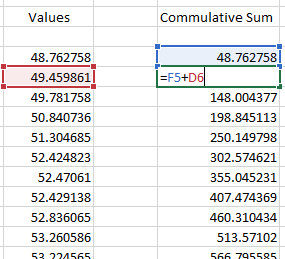# Error in taking Comulative_Sum with GH-Python

Hi Guys,

I am trying to get Cumulative Sum of multiple values (n) by using GH-Python as we do in excel, which results in a list of (n) values. But unfortunately, it is just calculating the sum of two consecutive values.

Can anyone help me with through this? I have attached both the excel screenshot and GH-Python Files.

Thanks

Cummulative_Problem.gh (6.5 KB)You are only ever adding two consecutive values from the same list in your Python code. You should instead do as you do with your spreadsheet: take the first value from the running total, the second value from the input list. Here an improved version of your script:

``````import rhinoscriptsyntax as rs
G=[]

# through using two lists. Not very good, since
# you have to juggle two indices into two separate
# lists, one of them is changing on each run
for G_i in range(-1, len(x)-1):
x_i = G_i+1
if G_i==-1: # check for index, to ensure we do what we need to
pt1 = 0
else:
pt1 = float(G[G_i])
pt2 = float(x[x_i])
sum = pt1+pt2
G.append(sum)
a=G
``````

A better approach is to just use a running total though. That takes a way the need of fiddling around with indices into two separate lists, and it is less code.

``````import rhinoscriptsyntax as rs
G=[]

# simple variable holding the sum as we move through the
# input list
running_total=0.0

for i in range(len(x)): # note no need for adjusted ranges
pt1 = float(x[i]) # nor having to index into two different lists
running_total = running_total + pt1
G.append(running_total)

a=G
``````
1 Like

Thanks a lot @nathanletwory for your guidance, It worked !